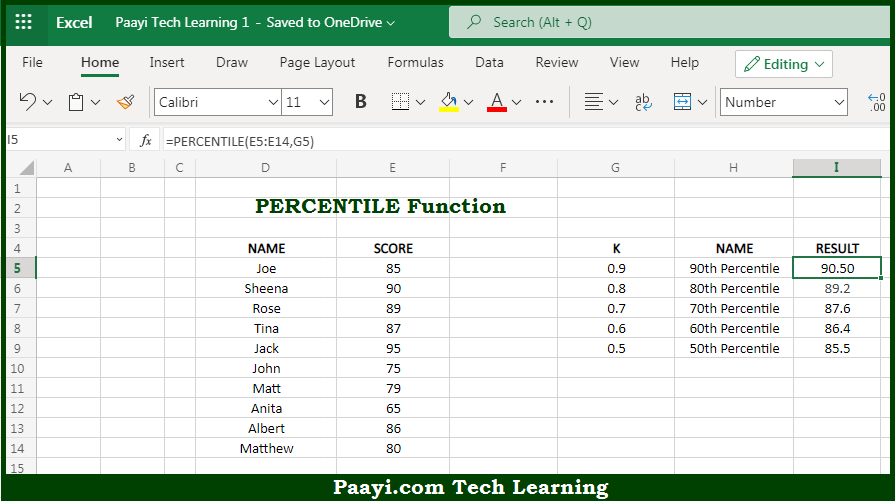# Learn How to Use Microsoft Excel PERCENTILE Function

Written by | 0 Comments | 629 Views

In this article, you will learn how to use the Microsoft Excel PERCENTILE function and its prime function in Microsoft Excel. You will also get to know the Microsoft Excel PERCENTILE function return value and syntax with the help of some examples.

Microsoft Excel PERCENTILE Function

The main purpose of the Microsoft Excel PERCENTILE function is to get the kth percentile. That implies, with the help of the PERCENTILE function you can able to calculates the "kth percentile" for the given set of data. It should be noted that percentile is a value below which a given percentage of values in a data set fall. You can also use PERCENTILE to determine the 90th percentile, the 80th percentile, and so on. So, with the help of the PERCENTILE function, you can able to get the kth percentile.

Return Value of PERCENTILE Function

The return value will be the calculated percentile for "k".

Syntax of PERCENTILE Function

=PERCENTILE(array, k)

Where the arguments:

• array: This is the data value array.
• k: This is the number representing the kth percentile.

## How to Use Microsoft Excel PERCENTILE Function?So we know that Microsoft Excel PERCENTILE function you can able to get the kth percentile. That implies, with the help of the PERCENTILE function you can able to calculates the "kth percentile" for the given set of data. It should be noted that percentile is a value below which a given percentage of values in a data set fall. You can also use PERCENTILE to determine the 90th percentile, the 80th percentile, and so on. So, with the help of the PERCENTILE function, you can able to get the kth percentile.